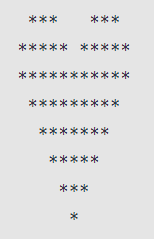Related Tags

javascript
communitycreator

# How to create a heart pattern using JavaScript

Ayush Trivedi

### Overview

In this shot, we’ll learn how to write a code and create a heart star pattern in this shot. This is a complex pattern.

The image below is the heart star pattern that we’re going to create.From the image, you can understand that it has two parts. The upper part is from lines 1 to 2, while the lower part is from lines 3 to 8.

### Code for the upper part

var n = 6;

for (let i = n / 2; i < n; i += 2) {
// print first spaces
for (let j = 1; j < n - i; j += 2) {
process.stdout.write(' ')
}
// print first stars
for (let j = 1; j < i + 1; j++) {
process.stdout.write('*')
}
// print second spaces
for (let j = 1; j < n - i + 1; j++) {
process.stdout.write(' ')
}
// print second stars
for (let j = 1; j < i + 1; j++) {
process.stdout.write('*')
}
console.log();
}
• Line 5: The first nested loop will print the spaces at the start. It will only print one space at the start.

• Line 9: The second nested loop will print the *. In the first iteration, it will print three stars, j < i+1, i=3, and j=1.

• Line 13: The third loop will print the gap spaces between the starts. In the first iteration, it will print three spaces, j < n - i + 1, n=6 , i=3, and j=1.

• Line 17: The fourth nested loop will print the *. It prints the remaining star. In the first iteration, it will print three stars, j < i+1, i=3, and j=1 just like the second nested loop.

### The final code

Finally, we need to write the code for the inverted pyramid after the code for the upper pattern.

var n = 6;

for (let i = n / 2; i < n; i += 2) {
// print first spaces
for (let j = 1; j < n - i; j += 2) {
process.stdout.write(' ')
}
// print first stars
for (let j = 1; j < i + 1; j++) {
process.stdout.write('*')
}
// print second spaces
for (let j = 1; j < n - i + 1; j++) {
process.stdout.write(' ')
}
// print second stars
for (let j = 1; j < i + 1; j++) {
process.stdout.write('*')
}
console.log();
}
// lower part
// inverted pyramid
for (let i = n; i > 0; i--) {
for (let j = 0; j < n - i; j++) {
process.stdout.write(' ')
}
for (let j = 1; j < i * 2; j++) {
process.stdout.write('*')
}
console.log();
}


### Explanation

We’ve already discussed the code from lines 1 to 21. Let’s see if we can create the lower half of the heart.

• Line 24: This is the outer for loop. It will do 5 iterations to complete the lower half of the heart.

• Line 25: The first nested for loop will print the spaces. In the first iteration, it will print zero spaces as j < n - i, n=6, i=6, and j=0.

• Line 28: The second nested for loop will print the *. In the first iteration, it will print eleven stars as j < i * 2, i=6, and j=1.

We have successfully created a heart shaped star pattern in the console.

RELATED TAGS

javascript
communitycreator

CONTRIBUTOR

Ayush Trivedi
RELATED COURSES

View all Courses

Keep Exploring

Learn in-demand tech skills in half the time